# Snap Cubes®

Snap Cubes are a versatile collection of 3⁄4-inch interlocking cubes which come in ten colors and connect on all six sides. They are pleasant to handle, easy to manipulate and, although simple in concept, can be used to develop a wide variety of mathematical ideas at many different levels of complexity. Since Snap Cubes come in 10 different colors, the cubes are useful for developing patterns, both one- and two-dimensional, based on color. The cubes can be arranged in a single layer to naturally fit into a square grid pattern, or they can be used to cover positions on a printed grid or game board. When the cubes are used to build three-dimensional structures, they lead naturally to the concepts of volume and surface area.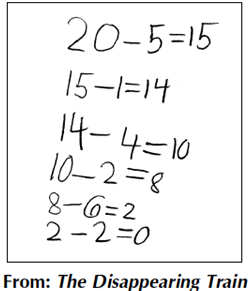The colors of the Snap Cubes can also be used to identify cubes in other contexts. For example, the different colors can represent designated quantities in various number situations. They become a sampling device when they are drawn from a bag, and they aid in concretely building bar graphs.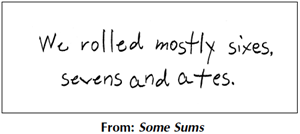Snap Cubes make natural and appealing counters. Since they snap together firmly, they are useful for young students as number models. If students build a stick (a group of cubes connected end to end) corresponding to each number from 1 to 10, it is natural for students to arrange them in a staircase and to talk about greater and less, longer and shorter. Numbers might also be represented by the following cube patterns, which are easily sorted into "even" ones, in which each cube is paired with another, and "odd" ones, in which there is an "odd man out."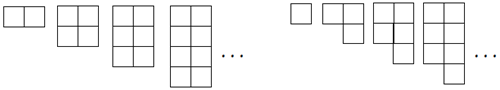Snap Cubes also help students to more easily see relationships such as the following: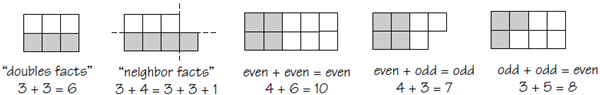Since there are large numbers of cubes in a set of Snap Cubes, they are useful for estimating and for developing number sense. Students can make a long stick with the cubes, estimate how many there are in the stick, and then separate it into sticks of 10, identify how many tens they have, and count the leftovers to find how many ones there are.

The colors of the cubes further make them useful in developing the concept of place value. Each color can represent a place value, and students can play exchange games in which, if they have 10 of one color, they can exchange them for one of the next color.

Snap Cubes are very suitable for developing an understanding of addition. They can be used as loose counters, with a different color for each addend. The colors can also broaden students’ understanding of subtraction. Students often think initially of subtraction as "take away." To act out 6 – 4, students put out 6 cubes and take away 4.

Snap Cubes are also ideal for developing the concept of multiplication, both as grouping and as an array. To show 3 × 4, students can make 3-inch sticks with 4 in each and count them all. Arranging these cubes in a rectangular array not only makes it visually easy to understand why 3 × 4 = 4 × 3 but also leads naturally into a model for understanding the formula for the area of a rectangle. In addition, Snap Cubes are suitable for exploring area, perimeter, volume, and surface area relations.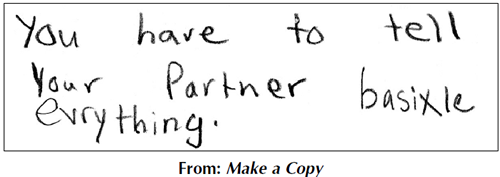Snap Cubes are a wonderful tool to use in helping students to represent numbers in terms of factors and to understand procedures for finding greatest common divisors and least common multiples. Snap Cubes are also a natural unit for measuring length, and using them can lead to early experience of ratio and proportion. Students can measure the same length in Snap Cubes and in another unit, perhaps inches. They can record their results for a few different lengths. They may then measure in just one unit and predict the measure in the other.

Snap Cubes are wonderful tools for assessing students’ mathematical thinking. Watching students work with their Snap Cubes gives you a sense of how they approach a mathematical problem. Their thinking can be "seen," in that thinking is expressed through the way they construct, recognize, and continue spatial patterns. When a class breaks up into small working groups, you are able to circulate, listen, and raise questions, all the while focusing on how individuals are thinking. Here is a perfect opportunity for authentic assessment.

Having students describe their structures and share their strategies and thinking with the whole class gives you another opportunity for observational assessment. Furthermore, you may want to gather students’ recorded work or invite them to choose pieces to add to their math portfolios.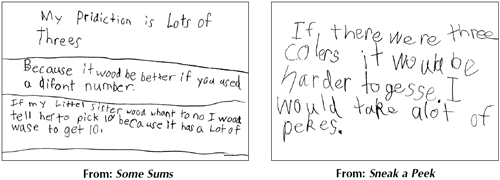## Lessons

Snap Cubes help children learn about whole numbers (counting, more and less, place value, addition, subtraction), measurement, probability, graphing, and geometry. They are ideal for exploring rhythmic patterns as well as growth patterns, and for doing spatial-reasoning tasks.

Here are two activities for getting to know Snap Cubes and to begin using them to learn and understand the math that they represent.

 Activity Curriculum Strands Topics Closest to 100In this game for two to four teams of two players each, children choose single Snap Cubes or sticks of 10 Snap Cubes according to the roll of a die. They position the cubes on a 100-grid in an effort to be the players to come closest to covering the grid. Number EstimationCountingAdditionPlace value PuzzlesChildren estimate, then find the number of Snap Cubes required to cover the areas of figures. MeasurementNumberGeometry CountingEstimationAreaComparing

Snap Cubes can be used to physically show how patterns operate and how equations balance out.

Here are two activities for getting to know Snap Cubes and to begin using them to learn and understand the math that they represent.

 Activity Curriculum Strands Topics A Tower of SquaresStudents use Snap Cubes to build larger and larger square prisms and stackthem to form a tower. They predict the numbers of cubes needed to producelarger squares and towers. GeometryNumberPatterns/Functions Square numbersCountingPattern recognitionVolume Plans and StructuresUsing Snap Cubes, students make structures and then create coded plans for their structures. Students exchange their structures and coded plans with other groups of students and match each structure with its coded plan. Geometry Spatial visualizationCongruence

Snap Cubes can be used to help students learn fraction, ratio and proportions, dedictive reasoning, algebra, and much, much more.

Here are two activities for getting to know Snap Cubes and to begin using them to learn and understand the math that they represent.

 Activity Curriculum Strands Topics Draw What You SeeStudents search to find all possible combinations of Snap Cubes that can be used to build shapes congruent with the yellow hexagon. Geometry Three-dimensional shapesPerspectiveVisual perception Pentacubes IIStudents search for different ways to arrange five Snap Cubes so that each arrangement, no matter how it is placed on a table, has at least one cube that does not touch the table. GeometryLogic Spatial visualizationCongruenceTransformational geometry

### Skills Taught

• Analyzing Data
• Area
• Chance
• Comparing
• Computation
• Congruence
• Counting Comparison
• Deductive Reasoning
• Equations
• Equivalence
• Equivalent Fractions
• Estimation
• Euler's Formula
• Experimental Probability
• Exponential Growth Sequence
• Exponential Notation
• Fairness
• Fibonacci Sequence
• Following Directions
• Game Strategies
• Greek Cross Numbers
• Growth Patterns
• Inequalities
• Interpreting Data
• Logic/Logical Reasoning
• Looking for Patterns
• Making and Testing Hypotheses
• Making Predictions
• Mental Math
• Multiples
• Nets
• Non-standard Measurements
• Number
• Number Sense
• Organizing and Interpreting Data
• Pattern Recognition
• Patterns/Functions
• Perimeter
• Permutations
• Perspective
• Probability/Statistics
• Proportion
• Pyramid Numbers
• Ratio
• Sampling
• Simulation
• Solid Geometry
• Spatial Visualization
• Square Numbers
• Surface Area
• Symmetry
• Theoretical Probability
• Three-dimensional Shapes
• Transformational Geometry
• Triangular Numbers
• Using Patterns
• Visual Perception
• Volume
• Writing Algebraic Expressions

### Subjects Taught

• Algebra
• Counting
• Division
• Fractions
• Geometry
• Measurement
• Multiplication
• Patterns
• Percents
• Place Value
• Sorting
• Subtraction

### Essential Manipulatives

View a selection of our competitively priced manipulatives.

### See the Research

Read a summary of the research in the white paper

Why Teach Mathematics with Manipulatives?